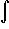#Interactive Real Analysis

Next | Previous | Glossary | Map

## 7.4. Lebesgue Integral

### Definition 7.4.5: Lebesgue Integral for Bounded Functions

Suppose f is a bounded function defined on a measurable set E with finite measure. Define the upper and lower Lebesgue integrals, respectively, as
I*(f)L = inf{s(x) dx: s is simple and sf }
I*(f)L = sup{s(x) dx: s is simple and sf }
If I*(f)L = I*(f)L the function f is called Lebesgue integrable over E and the Lebesgue integral of f over E is denoted byE f(x) dx
Next | Previous | Glossary | Map